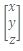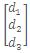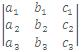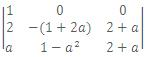Click to Chat

1800-1023-196

+91-120-4616500

CART 0

• 0

MY CART (5)

Use Coupon: CART20 and get 20% off on all online Study Material

ITEM
DETAILS
MRP
DISCOUNT
FINAL PRICE
Total Price: Rs.

There are no items in this cart.
Continue Shopping```System of Simultaneous Linear EquationsSystem of Simultaneous Linear Equations

Consider the following system of n linear equations in n unknowns:

a11 x1 + a12 x2 +.........+ a1n xn = d1

a21 x1 + a22 x2 +.........+ a2n xn = d2        .                              .   .

an1 x1 + an2 x2 +.........+ ann xn = dn

This system of equation can be written in the matrix form asor AX = D.

The n × n matrix A is called the coefficient matrix of the system of linear equations.

Homogeneous and Non-Homogeneous System of Linear Equations

A system of equations AX = D is called a homogeneous system if D = O. Otherwise it is called a non-homogeneous systems of equations.

Solution of a System of Equations

Consider the system of equation AX = D.

A set of values of the variables x1, x2, ......, xn which simultaneously satisfy all the equations is called a solution of the system of equations.

Consistent System

If the system of equations has one or more solutions, then it is said to be a consistent system of equations, otherwise it is an inconsistent system of equations.

Solution of a Non-Homogeneous System of Linear Equations

There are two methods of solving a non-homogeneous system of simultaneous linear equations.

(i)    Cramer's Rule

(ii)    Matrix Method

(i)     Cramer's Rule:

It is discussed under the topic of Determinants.

(ii)    Matrix Method:

Consider the equations

a1x + b1y + c1z = d1,

a2x + b2y + c2z = d2,                                                           ...... (i)

a3x + b3y + c3z = d3.

If A =X =and D =then the equation (i) is equivalent to the matrix equation

A X = D.                                                                            ...... (ii)

Multiplying both sides of (ii) by the inverse matrix A-1, we get

A-1 (AX) = A-1 D => IX = A-1D         [·.· A-1 A = I]

=> X = A-1 D =>...... (iii)

where A1, B1 etc. are the cofactors of a1, b2 etc. in the determinant

Δ =(Δ ≠ 0).

(i)     If A is a non-singular matrix, then the system of equations given by
AX = D has a unique solutions given by X = A-1 D.

(ii)    If A is a singular matrix, and (adjA)D = O, then the system of equations given by AX = D is consistent, with infinitely many solutions.

(iii)    If A is a singular matrix, and (adjA)D ≠ O, then the system of equation given by AX = D is inconsistent.

Solution of Homogeneous System of Linear Equations:

Let AX = O be a homogeneous system of n linear equation with n unknowns. Now if A is non-singular then the system of equations will have a unique solution i.e. trivial solution and if A is singular then the system of equations will have infinitely many solutions.

Illustration:

If the system of equations x + ay - z = 0, 2x - y + az = 0 and
ax + y + 2z = 0 has a non trivial solution, then find the value of 'a'.

Solution:

Using C2 → C2 - aC1, C3 → C3 + C1, we get

A == 0.

=> (2 + a)(-1 -2a - 1 + a2) = 0

=> (a + 2) (a2 - 2a - 2) = 0

=> a = -2, a = 1 + √3.

Illustration:

Find the value of 'k' for which the system of equations (k + 1)
x + 8y = 4k, kx + (k + 3)y = 3k -1 has no solution.

Solution:

For the system of equations to have no solution, we must have

(k+1)/k = 8/(k+3) ≠ 4k/(3k-1)

=> (k + 1) (k + 3) = 8k and 8 (3k - 1) ≠ 4k (k + 3)

=> k2 - 4k + 3 = 0 => k = 1, 3.

For  = 1, 8(3k - 1) = 16 and 4k (k + 3) = 16.

For k = 3, 8(3k - 1) = 64 and 4k (k + 3) = 72.

.·. for k = 3, 8(3k - 1) ≠ 4k (k + 3).

.·. k = 3 is the required value of 'k' for no solution.

To read more, Buy study materials of Matrices and Determinants comprising study notes, revision notes, video lectures, previous year solved questions etc. Also browse for more study materials on Mathematics here.

To know more about the study material of engineering and medical exams, please fill up the form given below:
```### Course Features

• 731 Video Lectures
• Revision Notes
• Previous Year Papers
• Mind Map
• Study Planner
• NCERT Solutions
• Discussion Forum
• Test paper with Video Solution